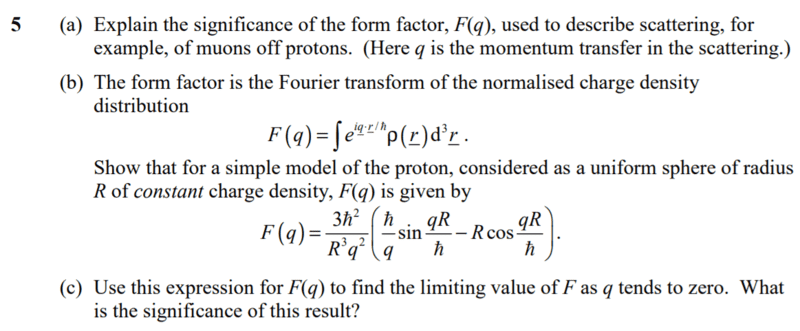# Form Factor for Scattering (like muons off of protons) (1 Viewer)

### Users Who Are Viewing This Thread (Users: 0, Guests: 1)

#### Martin89

1. The problem statement, all variables and given/known data2. Relevant equations
N/A

3. The attempt at a solution
I am trying to complete the last part of this question, part 5(c). My professor has told me that the form factor $$F(q)\rightarrow1$$ as $$q\rightarrow0$$ but I am unsure how to show this.

I believe that $$\lim_{{q }\rightarrow0} \frac{\sin\left( qRh/\hbar\right)}{q^3}=\infty,$$ and $$\lim_{{q }\rightarrow0} \frac{\cos\left( qRh/\hbar\right)}{q^2}=\infty.$$ Is someone able to please show me where I am going wrong? Thanks.

#### Attachments

• 149.7 KB Views: 145
Last edited by a moderator:

#### mjc123

Consider the term in brackets. Express sin x and cos x as power series in x, and evaluate this term as far as q2.

#### Martin89

Consider the term in brackets. Express sin x and cos x as power series in x, and evaluate this term as far as q2.
Thanks for the help. I discovered that I had to express sinx in powers up to q3 to solve correctly.

#### mjc123

Yes, but as the sin term is divided by q, the whole term in brackets goes up to q2. That's what I meant.
The key point is that if you treat the sin and cos terms separately, they both tend to infinity, but the whole term does not.

### The Physics Forums Way

We Value Quality
• Topics based on mainstream science
• Proper English grammar and spelling
We Value Civility
• Positive and compassionate attitudes
• Patience while debating
We Value Productivity
• Disciplined to remain on-topic
• Recognition of own weaknesses
• Solo and co-op problem solving